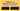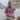# 5 Differences Between Arrow and Regular Functions

Posted May 16, 2020Start discussion

In JavaScript, you can define functions in many ways.

The first, usual way, is by using the `function` keyword:

``````// Function declaration
function greet(who) {
return `Hello, \${who}!`;
}``````
``````// Function expression
const greet = function(who) {
return `Hello, \${who}`;
}``````

The function declaration and function expression I’m going to reference as regular function.

The second way, available starting ES2015, is the arrow function syntax:

``````const greet = (who) => {
return `Hello, \${who}!`;
}``````

While both the regular and arrow syntaxes define functions, when would you choose one instead of another? That’s a good question.

In this post, I’m going to show the main differences between the two, so you could choose the right syntax for your needs.

## 1. this value

### 1.1 Regular function

Inside of a regular JavaScript function, `this` value (aka the execution context) is dynamic.

The dynamic context means that the value of `this` depends on how the function is invoked. In JavaScript, there are 4 ways you can invoke a regular function.

During a simple invocation the value of `this` equals to the global object (or `undefined` if the function runs in strict mode):

``````function myFunction() {
console.log(this);
}

// Simple invocation
myFunction(); // logs global object (window)``````

During a method invocation the value of `this` is the object owning the method:

``````const myObject = {
method() {
console.log(this);
}
};
// Method invocation
myObject.method(); // logs myObject``````

During an indirect invocation using `myFunc.call(thisVal, arg1, ..., argN)` or `myFunc.apply(thisVal, [arg1, ..., argN])` the value of `this` equals to the first argument:

``````function myFunction() {
console.log(this);
}

const myContext = { value: 'A' };

myFunction.call(myContext);  // logs { value: 'A' }
myFunction.apply(myContext); // logs { value: 'A' }``````

During a constructor invocation using `new` keyword `this` equals to the newly created instance:

``````function MyFunction() {
console.log(this);
}

new MyFunction(); // logs an instance of MyFunction``````

### 1.2 Arrow function

The behavior of `this` inside of an arrow function differs considerably from the regular function’s `this` behavior.

No matter how or where being executed, `this` value inside of an arrow function always equals `this` value from the outer function. In other words, the arrow function resolves `this` lexically. In another words, the arrow function doesn’t define its own execution context.

In the following example, `myMethod()` is an outer function of `callback()` arrow function:

``````const myObject = {
myMethod(items) {
console.log(this); // logs myObject    const callback = () => {
console.log(this); // logs myObject    };
items.forEach(callback);
}
};

myObject.myMethod([1, 2, 3]); ``````

`this` value inside the arrow function `callback()` equals to `this` of the outer function `myMethod()`.

`this` resolved lexically is one of the great features of arrow functions. When using callbacks inside methods you are sure the arrow function doesn’t define its own `this`: no more `const self = this` or `callback.bind(this)` workarounds.

Contrary to a regular function, the indirect invocation of an arrow function using `myArrowFunc.call(thisVal)` or `myArrowFunc.apply(thisVal)` doesn’t change the value of `this`: the context value is always resolved lexically.

## 2. Constructors

### 2.1 Regular function

As seen in the previous section, the regular function can easily construct objects.

For example, the `Car()` function creates instances of a car:

``````function Car(color) {
this.color = color;
}

const redCar = new Car('red');
redCar instanceof Car; // => true``````

`Car` is a regular function, and when invoked with `new` keyword, it creates new instances of `Car` type.

### 2.2 Arrow function

A consequence of `this` resolved lexically is that an arrow function cannot be used as a constructor.

If you try to invoke an arrow function prefixed with `new` keyword, JavaScrip throws an error:

``````const Car = (color) => {
this.color = color;
};

const redCar = new Car('red'); // TypeError: Car is not a constructor ``````

Invoking `new Car('red')`, where `Car` is an arrow function, throws `TypeError: Car is not a constructor`.

## 3. arguments object

### 3.1 Regular function

Inside the body of a regular function, `arguments` is a special array-like object containing the list of arguments with which the function has been invoked.

Let’s invoke `myFunction` function with 2 arguments:

``````function myFunction() {
console.log(arguments);}

myFunction('a', 'b'); // logs { 0: 'a', 1: 'b'}``````

`arguments` array-like object contains the invocation arguments: `'a'` and `'b'`.

### 3.2 Arrow function

On the other side, no `arguments` special keyword is defined inside an arrow function.

Again (same as with `this` value), the `arguments` object is resolved lexically: the arrow function accesses `arguments` from the outer function.

Let’s try to access `arguments` inside of an arrow function:

``````function myRegularFunction() {
const myArrowFunction = () => {    console.log(arguments);  }
myArrowFunction('c', 'd');
}

myRegularFunction('a', 'b'); // logs { 0: 'a', 1: 'b' }``````

The arrow function `myArrowFunction()` is invoked with the arguments `'c'`, `'d'`. Still, inside of its body, `arguments` object equals to the arguments of `myRegularFunction()` invocation: `'a'`, `'b'`.

If you’d like to access the direct arguments of the arrow function, then you can use the rest parameters feature:

``````function myRegularFunction() {
const myArrowFunction = (...args) => {    console.log(args);  }
myArrowFunction('c', 'd');
}

myRegularFunction('a', 'b'); // logs { 0: 'c', 1: 'd' }``````

`...args` rest parameter collects the execution arguments of the arrow function: `{ 0: 'c', 1: 'd' }`.

## 4. Implicit return

### 4.1 Regular function

Just use `return expression` statement to return a result from a function:

``````function myFunction() {
return 42;
}

myFunction(); // => 42``````

If the `return` statement is missing, or there’s no expression after return statement, the regular function implicitely returns `undefined`:

``````function myEmptyFunction() {
42;
}

function myEmptyFunction2() {
42;
return;
}

myEmptyFunction();  // => undefined
myEmptyFunction2(); // => undefined``````

### 4.2 Arrow function

You can return values from the arrow function the same way as from a regular function, but with one useful exception.

If the arrow function contains one expression, and you omit the function’s curly braces, then the expression is implicitely returned. These are the inline arrows function.

``````const increment = (num) => num + 1;

increment(41); // => 42``````

The `increment()` arrow consists of only one expression: `num + 1`. This expression is implicitly returned by the arrow function without the use of `return` keyword.

## 5. Methods

### 5.1 Regular function

The regular functions are the usual way to define methods on classes.

In the following class `Hero`, the method `logName()` is defined using a regular function:

``````class Hero {
constructor(heroName) {
this.heroName = heroName;
}

logName() {    console.log(this.heroName);  }}

const batman = new Hero('Batman');``````

Usually, the regular functions as methods are the way to go.

Sometimes you’d need to supply the method as a callback, for example to `setTimeout()` or an event listener. In such cases, you might encounter difficulties to access `this` value.

For example, let’s use use `logName()` method as a callback to `setTimeout()`:

``````setTimeout(batman.logName, 1000);
// after 1 second logs "undefined"``````

After 1 second, `undefined` is logged to console. `setTimeout()` performs a simple invocation of `logName` (where `this` is the global object). That’s when the method is separated from the object.

Let’s bind `this` value manually to the right context:

``````setTimeout(batman.logName.bind(batman), 1000);
// after 1 second logs "Batman"``````

`batman.logName.bind(batman)` binds `this` value to `batman` instance. Now you’re sure that the method doesn’t lose the context.

Binding `this` manually requires boilerplate code, especially if you have lots of methods. There’s a better way: the arrow functions as a class field.

### 5.2 Arrow function

Thanks to Class fields proposal (at this moment at stage 3) you can use the arrow function as methods inside classes.

Now, in contrast with regular functions, the method defined using an arrow binds `this` lexically to the class instance.

Let’s use the arrow function as a field:

``````class Hero {
constructor(heroName) {
this.heroName = heroName;
}

logName = () => {    console.log(this.heroName);  }}

const batman = new Hero('Batman');``````

Now you can use `batman.logName` as a callback without any manual binding of `this`. The value of `this` inside `logName()` method is always the class instance:

``````setTimeout(batman.logName, 1000);
// after 1 second logs "Batman"``````

## 6. Summary

Understanding the differences between regular and arrow functions helps choose the right syntax for specific needs.

`this` value inside a regular function is dynamic and depends on the invocation. But `this` inside the arrow function is bound lexically and equals to `this` of the outer function.

`arguments` object inside the regular functions contains the list of arguments. The arrow function, on the opposite, doesn’t define `arguments` (but you can easily access the arrow function arguments using a rest parameter `...args`).

If the arrow function has one expression, then the expression is returned implicitly, even without using the `return` keyword.

Last but not least, you can define methods using the arrow function syntax inside classes. Fat arrow methods bind `this` value to the class instance.

Anyhow the fat arrow method is invoked, `this` always equals to the class instance, which is useful when the methods are used as callbacks.

What other differences between arrow and regular functions do you know?

## Quality posts into your inbox

I regularly publish posts containing:

• Important JavaScript concepts explained in simple words
• Overview of new JavaScript features
• How to use TypeScript and typing
• Software design and good coding practices